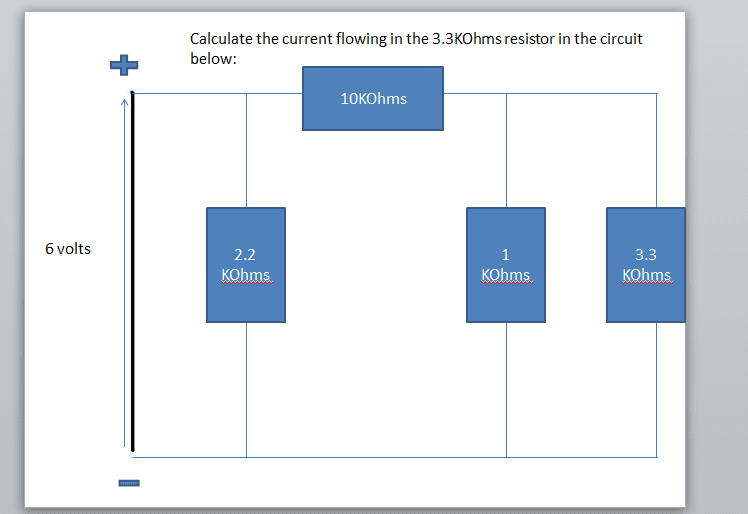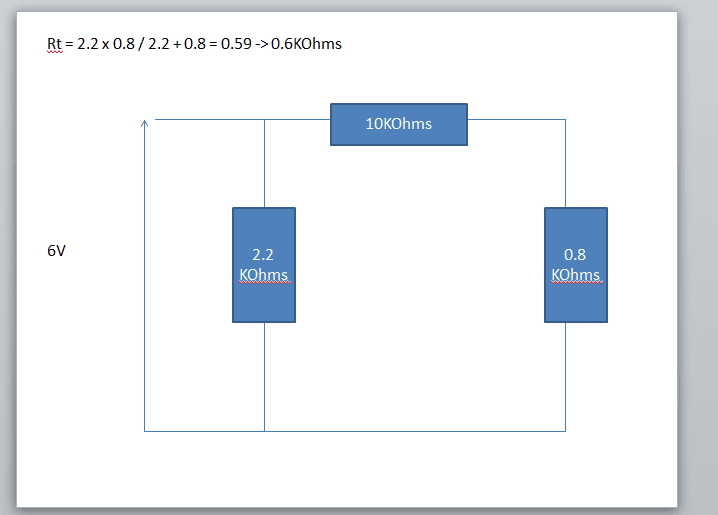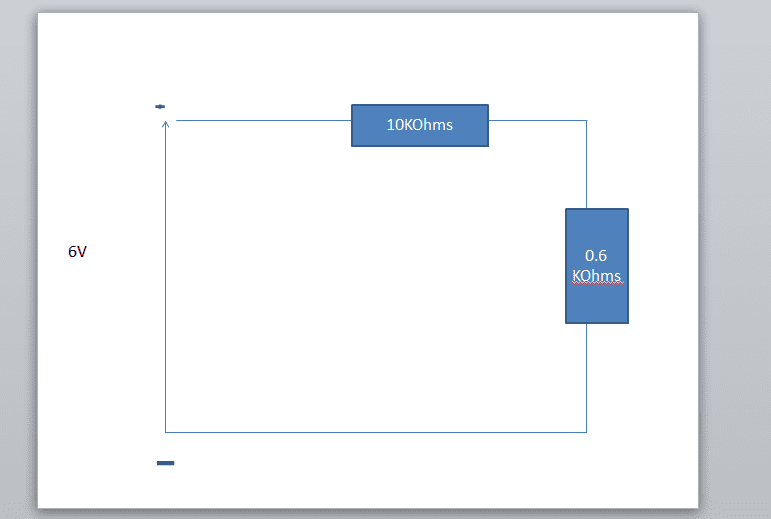# Current flowing through the resistor

joaofbi1
I'm just stuck on a question that I've been given as a quick test, it's really stumped me. I was wondering if anyone could assist me.

Here's the question:Thank you.

Staff Emeritus
Hi joaofbi1. http://img96.imageshack.us/img96/5725/red5e5etimes5e5e45e5e25.gif [Broken]

Let's see your thoughts on how to solve this. Then we can see where you may be going wrong.First step, draw in some current paths so you can see where the current is flowing.

Last edited by a moderator:
Gold Member
You can solve this via mesh analysis: write the currents I1,I2,I3 as loops passing around each of the three independent loops, the write the three mesh equations, taking proper care of the directions which you assigned (I used clockwise for all three) when summing the currents from shared resistors.

6V = 2.2k(I1-I2)
0 = 2.2k(I2-I1) + 10k(I2) + 1k(I2-I3)
0 = 1k(I3-I2) + 3.3k(I3)

Then solve the three simultaneous linear equations via your favorite method (substitution, elimination, Cramer's rule), and I3 is your answer.

I also considered a Delta-Y transform but it didn't seem to make the solution go any faster.

joaofbi1
Firstly as 1KΩ and 3.3Ω are parallel I used the formula:
Rt= R1XR2 / R1 + R2 so it would've been Rt = 1x3.3 / 1 + 3.3 which gave me the answer of 0.8kΩ

After that I noticed that 2.2kΩ, 10kΩ and 0.8kΩ was kind of in a circuit, if you rearranged it, because of that I used the formula:
Rt = R1 + R2 + R3 which would have been Rt= 2.2 + 10 + 0.8 which gave me 13kΩ

After that I used the formula I= V/R - But before that I converted 13kΩ into Ω by ten to the power of -3 so I got 13x10(-3).
After that I did Voltage / Resistance -> 6/(13x10(-3)) which gave me the current of 462Amps which then I converted into mA and got 0.462mA.

That's where I got to, now I'm stuck.

joaofbi1
You can solve this via mesh analysis: write the currents I1,I2,I3 as loops passing around each of the three independent loops, the write the three mesh equations, taking proper care of the directions which you assigned (I used clockwise for all three) when summing the currents from shared resistors.

6V = 2.2k(I1-I2)
0 = 2.2k(I2-I1) + 10k(I2) + 1k(I2-I3)
0 = 1k(I3-I2) + 3.3k(I3)

Then solve the three simultaneous linear equations via your favorite method (substitution, elimination, Cramer's rule), and I3 is your answer.

I also considered a Delta-Y transform but it didn't seem to make the solution go any faster.

I've just started the electronic course so I'm quite new to the method that you're using. So I don't quite understand it.

Gold Member
I agree that the parallel combination of 3.3k and 1k is ~0.8k (I get 0.77k). But this doesn't help you to get the current through the 3.3k resistor!

Also you cannot sum the resistors around the middle loop ... this is only valid for resistors in series; resistors in series appear 1-2-3 along the same piece of conductor without any additional branches. In this case you have an additional branch which has the 6V voltage source.

It is possible to continue the reduction of the resistor network into a single loop with the voltage source plus one resistor in series. This would provide the total current being supplied by the battery, but now you have lost track of the 3.3k resistor!

The easiest way is to apply the Kirchoff voltage law (KVL) around each loop, or the Kirchoff current law (KCL) at each node, or apply a method (like the mesh method) which is derived from those laws.

Gold Member
Are you self-study, or is this a course with an instructor?

You know Ohm's law; the next step is usually to teach KVL and KCL followed by some practice.

The mesh method is simply a faster, systematic application of KVL; nodal analysis is a faster, systematic application of KCL. In this case I used the mesh method; you can arrive at the same results via KVL or KCL though the equations may have a different appearance ... but the three currents will be the same.

joaofbi1
I've not learned KVL nor KCL yet, and yes this course is with an instructor. I've been told that I have to find the current around the whole circuit before calculating the current through the given resistor hence the reason why I have attempted to calculate the total resistance across the whole circuit.

Is this not possible to do with Ohm's law? If it is then could you help me out using Ohm's law please?

Gold Member
Yes, you solve for the total current by reducing the resistor network to an equivalent single resistor. You have already started on this by combining the two resistors that were in parallel.

If you now redraw the circuit with the reduction (using the 0.8 kOhm resistor) you should see that there is now a series combination of two resistors which can be combined; do this, and redraw the circuit.

This time you should see two resistors in parallel with the battery. You can combine the two resistors and use Ohm's law to find the total current.

Do that, keeping all of the circuit diagrams ... then you can start solving backwards, but first things first!

•1 person
joaofbi1
So firstly I do this:then it turns into this:Right? Then what shall I do, this is the bit where I get confused on what to do with the two resistors.

Staff Emeritus
I suggested that you should draw some current paths, to help with the analysis, for a good reason.

Keep it as 3 resistors, 2.2, 10 and 0.8. Draw some current paths, these being shown as closed loops.

joaofbi1
I suggested that you should draw some current paths, to help with the analysis, for a good reason.

Keep it as 3 resistors, 2.2, 10 and 0.8. Draw some current paths, these being shown as closed loops.

What do you mean by draw circuit paths?

Gold Member
The 10k and 0.8k resistors are in series; the 2.2k is NOT in parallel with the 0.8k resistor because there is something on the upper bus between them: the 10k resistor.

So combine the 10k with the 0.8k in series: 10.8k, and then you are left with two resistors in parallel, the 2.2k and the 10.8k. Combine them and then solve for the total current.

joaofbi1
The 10k and 0.8k resistors are in series; the 2.2k is NOT in parallel with the 0.8k resistor because there is something on the upper bus between them: the 10k resistor.

So combine the 10k with the 0.8k in series: 10.8k, and then you are left with two resistors in parallel, the 2.2k and the 10.8k. Combine them and then solve for the total current.

Oh I understand what you mean now, so once I've found the total current what formula do I use to find the current going through the resistor?

Gold Member
Now you will have to use your sequence of diagrams in reverse, solving for the current flow through each branch.

For the first step you will have two resistors in parallel; part of the total current will pass through each branch.

Since one of the resistors is the 2.2k resistor from the original circuit, you can go back and label both the total current drawn from the battery, and the current passing through the 2.2k resistor. The remaining current must pass through the 10k resistor.

What will then happen to this part of the current?

joaofbi1
I got the total current across the whole circuit of 3.3. So the voltage across the 2.2 resistor would be 3.3 X 2.2? But how would that help me find the 3.3 value?

Gold Member
You have two resistors in parallel on this diagram ... the next to last circuit ... one is 2.2k, the other is 10.8k.

They both see the same voltage: 6 volts. So you can calculate the current through each of these two paths via Ohm's law. You need to do this so that you know the current going through the 10.8k equivalent resistor.

You will use that current for the next step.

I get 1.98k (2k) for the total equivalent resistance, which means that the total current is
I=6v/2k Ohms=3 mAmps. You should always keep track of your units.

Correction: I get 1.83 kOhms for the equivalent resistance, and 3.3 mAmps for the total current - so your total current is correct.

Last edited:
joaofbi1
So once I've calculated the current through the 2.2 and 10 resistor then what calculation would I use?

Gold Member
You want the current through the 2.2k and the 10.8k ohm resistors. They should sum to 3.3mA.

The current through the 10.8 kOhm resistor will then be the same as the current through the 10 kOhm resistor in the original circuit: it will then divide as it goes through the original two parallel resistors, the 1k and the 3.3k ohm resistors.

You have two ways to solve this final step.

joaofbi1
So once I get the current for the 10.8 resistor do I divide that current by 1and 3.3

Staff Emeritus
So once I get the current for the 10.8 resistor do I divide that current by 1and 3.3
Not exactly.

But you could determine how much of the battery's voltage is "lost" because of the 10kΩ resistor, so the remainder is what appears across the pair of parallel resistors.

joaofbi1
Im quite confused, what calculations would I need to perform?

Staff Emeritus
Im quite confused, what calculations would I need to perform?
How many volts are lost across the 10k resistor? Use Ohms Law.

joaofbi1
Im not at home yet so I cannot answer that. But after I see how much current the 10ohms resistor takes then do I use the current amount of current I have after the 10kohmd resistor and use the same calculation on the 3.3 resistor?

joaofbi1
Ok so I'm home, so this is what was calculated 3.3A x 10KOhms = 33Volts through the 10kOhms resistor. Then what do I do after that operation?

Gold Member
It should be 3.3 mA through 10k ohms. Pay attention to your units!

And that is the total current; we previously calculated the current for this branch - what is it?

Last edited:
joaofbi1
So how do I calculate how much mA I've lost through the resistor? What formula?

Gold Member
The second to last circuit had two parallel resistors: 2.2k and 10.8 k. Each sees 6 V, so you can apply Ohm's law to each resistor.

I1 = 6 V/2.2k ohms = 2.73 mA; I2 = 6 V/10.8 k ohms = 0.55 mA. These add up to 3.3 mA total current which we obtained from the final circuit.

So the current through the 10 k ohm resistor will be I2 = 0.55 mA.

This is the current that will be available to the parallel resistors 1 k and 3.3 k ohm; when they were combined they provided the addition 0.8 k ohms that was combined with the 10 k resistor in series to obtain the 10.8 k ohm equivalent resistor.

This process of working backwards, and marking the resulting currents on each as we walked backwards up the circuits has provided all of the information needed.

joaofbi1
It should be 3.3 mA through 10k ohms. Pay attention to your units!

And that is the total current; we previously calculated the current for this branch - what is it?

So how do I Calculate how much mA I have lost through the resistor?

Gold Member
Note that the voltage seen by these parallel resistors is the same, and can be found as V= 6 V - 10k Ohm x 0.55 mA = 6 - 5.5 [V] = 0.5 V.

Or we could have solved from the equivalent resistance of the parallel resistors which was 770 Ohms, with a current of 0.55 mA gives V = 0.770 kOhms x 0.55 mA = 0.44 [V]

These voltages are close (0.5, 0.44) but we should get the same answer both ways ... this indicates that there is an error earlier in the calculations which you should recheck.

Assuming that 0.5 V is correct you can use Ohm's law to get the current I3 = 0.5 V / 3.3 k Ohms = 0.15 mA.

Gold Member
So how do I Calculate how much mA I have lost through the resistor?

No current is lost through a resistor; only the voltage is used up.

joaofbi1
But where exactly did you get 0.55mA from? That has completely stumped me.